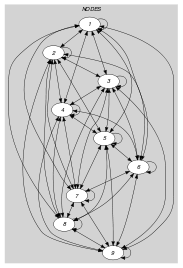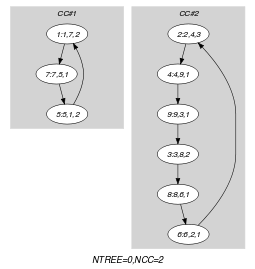## 5.104. cycle_card_on_path

Origin

CHIP

Constraint

$\mathrm{𝚌𝚢𝚌𝚕𝚎}_\mathrm{𝚌𝚊𝚛𝚍}_\mathrm{𝚘𝚗}_\mathrm{𝚙𝚊𝚝𝚑}\left(\mathrm{𝙽𝙲𝚈𝙲𝙻𝙴},\mathrm{𝙽𝙾𝙳𝙴𝚂},\mathrm{𝙰𝚃𝙻𝙴𝙰𝚂𝚃},\mathrm{𝙰𝚃𝙼𝙾𝚂𝚃},\mathrm{𝙿𝙰𝚃𝙷}_\mathrm{𝙻𝙴𝙽},\mathrm{𝚅𝙰𝙻𝚄𝙴𝚂}\right)$

Arguments
 $\mathrm{𝙽𝙲𝚈𝙲𝙻𝙴}$ $\mathrm{𝚍𝚟𝚊𝚛}$ $\mathrm{𝙽𝙾𝙳𝙴𝚂}$ $\mathrm{𝚌𝚘𝚕𝚕𝚎𝚌𝚝𝚒𝚘𝚗}\left(\mathrm{𝚒𝚗𝚍𝚎𝚡}-\mathrm{𝚒𝚗𝚝},\mathrm{𝚜𝚞𝚌𝚌}-\mathrm{𝚍𝚟𝚊𝚛},\mathrm{𝚌𝚘𝚕𝚘𝚞𝚛}-\mathrm{𝚍𝚟𝚊𝚛}\right)$ $\mathrm{𝙰𝚃𝙻𝙴𝙰𝚂𝚃}$ $\mathrm{𝚒𝚗𝚝}$ $\mathrm{𝙰𝚃𝙼𝙾𝚂𝚃}$ $\mathrm{𝚒𝚗𝚝}$ $\mathrm{𝙿𝙰𝚃𝙷}_\mathrm{𝙻𝙴𝙽}$ $\mathrm{𝚒𝚗𝚝}$ $\mathrm{𝚅𝙰𝙻𝚄𝙴𝚂}$ $\mathrm{𝚌𝚘𝚕𝚕𝚎𝚌𝚝𝚒𝚘𝚗}\left(\mathrm{𝚟𝚊𝚕}-\mathrm{𝚒𝚗𝚝}\right)$
Restrictions
 $\mathrm{𝙽𝙲𝚈𝙲𝙻𝙴}\ge 1$ $\mathrm{𝙽𝙲𝚈𝙲𝙻𝙴}\le |\mathrm{𝙽𝙾𝙳𝙴𝚂}|$ $\mathrm{𝚛𝚎𝚚𝚞𝚒𝚛𝚎𝚍}$$\left(\mathrm{𝙽𝙾𝙳𝙴𝚂},\left[\mathrm{𝚒𝚗𝚍𝚎𝚡},\mathrm{𝚜𝚞𝚌𝚌},\mathrm{𝚌𝚘𝚕𝚘𝚞𝚛}\right]\right)$ $\mathrm{𝙽𝙾𝙳𝙴𝚂}.\mathrm{𝚒𝚗𝚍𝚎𝚡}\ge 1$ $\mathrm{𝙽𝙾𝙳𝙴𝚂}.\mathrm{𝚒𝚗𝚍𝚎𝚡}\le |\mathrm{𝙽𝙾𝙳𝙴𝚂}|$ $\mathrm{𝚍𝚒𝚜𝚝𝚒𝚗𝚌𝚝}$$\left(\mathrm{𝙽𝙾𝙳𝙴𝚂},\mathrm{𝚒𝚗𝚍𝚎𝚡}\right)$ $\mathrm{𝙽𝙾𝙳𝙴𝚂}.\mathrm{𝚜𝚞𝚌𝚌}\ge 1$ $\mathrm{𝙽𝙾𝙳𝙴𝚂}.\mathrm{𝚜𝚞𝚌𝚌}\le |\mathrm{𝙽𝙾𝙳𝙴𝚂}|$ $\mathrm{𝙰𝚃𝙻𝙴𝙰𝚂𝚃}\ge 0$ $\mathrm{𝙰𝚃𝙻𝙴𝙰𝚂𝚃}\le \mathrm{𝙿𝙰𝚃𝙷}_\mathrm{𝙻𝙴𝙽}$ $\mathrm{𝙰𝚃𝙼𝙾𝚂𝚃}\ge \mathrm{𝙰𝚃𝙻𝙴𝙰𝚂𝚃}$ $\mathrm{𝙿𝙰𝚃𝙷}_\mathrm{𝙻𝙴𝙽}\ge 0$ $|\mathrm{𝚅𝙰𝙻𝚄𝙴𝚂}|\ge 1$ $\mathrm{𝚛𝚎𝚚𝚞𝚒𝚛𝚎𝚍}$$\left(\mathrm{𝚅𝙰𝙻𝚄𝙴𝚂},\mathrm{𝚟𝚊𝚕}\right)$ $\mathrm{𝚍𝚒𝚜𝚝𝚒𝚗𝚌𝚝}$$\left(\mathrm{𝚅𝙰𝙻𝚄𝙴𝚂},\mathrm{𝚟𝚊𝚕}\right)$
Purpose

Consider a digraph $G$ described by the $\mathrm{𝙽𝙾𝙳𝙴𝚂}$ collection. $\mathrm{𝙽𝙲𝚈𝙲𝙻𝙴}$ is the number of circuits for covering $G$ in such a way that each vertex belongs to a single circuit. In addition the following constraint must also hold: on each set of $\mathrm{𝙿𝙰𝚃𝙷}_\mathrm{𝙻𝙴𝙽}$ consecutive distinct vertices of each final circuit, the number of vertices for which the attribute colour takes his value in the collection of values $\mathrm{𝚅𝙰𝙻𝚄𝙴𝚂}$ should be located within the range $\left[\mathrm{𝙰𝚃𝙻𝙴𝙰𝚂𝚃},\mathrm{𝙰𝚃𝙼𝙾𝚂𝚃}\right]$.

Example
$\left(\begin{array}{c}2,〈\begin{array}{ccc}\mathrm{𝚒𝚗𝚍𝚎𝚡}-1\hfill & \mathrm{𝚜𝚞𝚌𝚌}-7\hfill & \mathrm{𝚌𝚘𝚕𝚘𝚞𝚛}-2,\hfill \\ \mathrm{𝚒𝚗𝚍𝚎𝚡}-2\hfill & \mathrm{𝚜𝚞𝚌𝚌}-4\hfill & \mathrm{𝚌𝚘𝚕𝚘𝚞𝚛}-3,\hfill \\ \mathrm{𝚒𝚗𝚍𝚎𝚡}-3\hfill & \mathrm{𝚜𝚞𝚌𝚌}-8\hfill & \mathrm{𝚌𝚘𝚕𝚘𝚞𝚛}-2,\hfill \\ \mathrm{𝚒𝚗𝚍𝚎𝚡}-4\hfill & \mathrm{𝚜𝚞𝚌𝚌}-9\hfill & \mathrm{𝚌𝚘𝚕𝚘𝚞𝚛}-1,\hfill \\ \mathrm{𝚒𝚗𝚍𝚎𝚡}-5\hfill & \mathrm{𝚜𝚞𝚌𝚌}-1\hfill & \mathrm{𝚌𝚘𝚕𝚘𝚞𝚛}-2,\hfill \\ \mathrm{𝚒𝚗𝚍𝚎𝚡}-6\hfill & \mathrm{𝚜𝚞𝚌𝚌}-2\hfill & \mathrm{𝚌𝚘𝚕𝚘𝚞𝚛}-1,\hfill \\ \mathrm{𝚒𝚗𝚍𝚎𝚡}-7\hfill & \mathrm{𝚜𝚞𝚌𝚌}-5\hfill & \mathrm{𝚌𝚘𝚕𝚘𝚞𝚛}-1,\hfill \\ \mathrm{𝚒𝚗𝚍𝚎𝚡}-8\hfill & \mathrm{𝚜𝚞𝚌𝚌}-6\hfill & \mathrm{𝚌𝚘𝚕𝚘𝚞𝚛}-1,\hfill \\ \mathrm{𝚒𝚗𝚍𝚎𝚡}-9\hfill & \mathrm{𝚜𝚞𝚌𝚌}-3\hfill & \mathrm{𝚌𝚘𝚕𝚘𝚞𝚛}-1\hfill \end{array}〉,1,2,3,\hfill \\ 〈1〉\hfill \end{array}\right)$

The constraint $\mathrm{𝚌𝚢𝚌𝚕𝚎}_\mathrm{𝚌𝚊𝚛𝚍}_\mathrm{𝚘𝚗}_\mathrm{𝚙𝚊𝚝𝚑}$ holds since the vertices of the $\mathrm{𝙽𝙾𝙳𝙴𝚂}$ collection correspond to a set of disjoint circuits and since, for each set of 3 (i.e., $\mathrm{𝙿𝙰𝚃𝙷}_\mathrm{𝙻𝙴𝙽}=3$) consecutive vertices, colour 1 (i.e., the value provided by the $\mathrm{𝚅𝙰𝙻𝚄𝙴𝚂}$ collection) occurs at least once (i.e., $\mathrm{𝙰𝚃𝙻𝙴𝙰𝚂𝚃}=1$) and at most twice (i.e., $\mathrm{𝙰𝚃𝙼𝙾𝚂𝚃}=2$).

Typical
 $|\mathrm{𝙽𝙾𝙳𝙴𝚂}|>2$ $\mathrm{𝙽𝙲𝚈𝙲𝙻𝙴}<|\mathrm{𝙽𝙾𝙳𝙴𝚂}|$ $\mathrm{𝙰𝚃𝙻𝙴𝙰𝚂𝚃}<\mathrm{𝙿𝙰𝚃𝙷}_\mathrm{𝙻𝙴𝙽}$ $\mathrm{𝙰𝚃𝙼𝙾𝚂𝚃}>0$ $\mathrm{𝙿𝙰𝚃𝙷}_\mathrm{𝙻𝙴𝙽}>1$ $|\mathrm{𝙽𝙾𝙳𝙴𝚂}|>|\mathrm{𝚅𝙰𝙻𝚄𝙴𝚂}|$ $\mathrm{𝙰𝚃𝙻𝙴𝙰𝚂𝚃}>0\vee \mathrm{𝙰𝚃𝙼𝙾𝚂𝚃}<\mathrm{𝙿𝙰𝚃𝙷}_\mathrm{𝙻𝙴𝙽}$
Symmetries
• Items of $\mathrm{𝙽𝙾𝙳𝙴𝚂}$ are permutable.

• An occurrence of a value of $\mathrm{𝙽𝙾𝙳𝙴𝚂}.\mathrm{𝚌𝚘𝚕𝚘𝚞𝚛}$ that belongs to $\mathrm{𝚅𝙰𝙻𝚄𝙴𝚂}.\mathrm{𝚟𝚊𝚕}$ (resp. does not belong to $\mathrm{𝚅𝙰𝙻𝚄𝙴𝚂}.\mathrm{𝚟𝚊𝚕}$) can be replaced by any other value in $\mathrm{𝚅𝙰𝙻𝚄𝙴𝚂}.\mathrm{𝚟𝚊𝚕}$ (resp. not in $\mathrm{𝚅𝙰𝙻𝚄𝙴𝚂}.\mathrm{𝚟𝚊𝚕}$).

• $\mathrm{𝙰𝚃𝙻𝙴𝙰𝚂𝚃}$ can be decreased to any value $\ge 0$.

• $\mathrm{𝙰𝚃𝙼𝙾𝚂𝚃}$ can be increased.

• Items of $\mathrm{𝚅𝙰𝙻𝚄𝙴𝚂}$ are permutable.

Usage

Assume that the vertices of $G$ are partitioned into the following two categories:

• Clients to visit.

• Depots where one can reload a vehicle.

Using the $\mathrm{𝚌𝚢𝚌𝚕𝚎}_\mathrm{𝚌𝚊𝚛𝚍}_\mathrm{𝚘𝚗}_\mathrm{𝚙𝚊𝚝𝚑}$ constraint we can express a constraint like: after visiting three consecutive clients we should visit a depot. This is typically not possible with the $\mathrm{𝚊𝚝𝚖𝚘𝚜𝚝}$ constraint since we do not know in advance the set of variables involved in the $\mathrm{𝚊𝚝𝚖𝚘𝚜𝚝}$ constraint.

Remark

This constraint is a special case of the $\mathrm{𝚜𝚎𝚚𝚞𝚎𝚗𝚌𝚎}$ parameter of the $\mathrm{𝚌𝚢𝚌𝚕𝚎}$ constraint of

Keywords
Arc input(s)

$\mathrm{𝙽𝙾𝙳𝙴𝚂}$

Arc generator
$\mathrm{𝐶𝐿𝐼𝑄𝑈𝐸}$$↦\mathrm{𝚌𝚘𝚕𝚕𝚎𝚌𝚝𝚒𝚘𝚗}\left(\mathrm{𝚗𝚘𝚍𝚎𝚜}\mathtt{1},\mathrm{𝚗𝚘𝚍𝚎𝚜}\mathtt{2}\right)$

Arc arity
Arc constraint(s)
$\mathrm{𝚗𝚘𝚍𝚎𝚜}\mathtt{1}.\mathrm{𝚜𝚞𝚌𝚌}=\mathrm{𝚗𝚘𝚍𝚎𝚜}\mathtt{2}.\mathrm{𝚒𝚗𝚍𝚎𝚡}$
Graph property(ies)
 $•$$\mathrm{𝐍𝐓𝐑𝐄𝐄}$$=0$ $•$$\mathrm{𝐍𝐂𝐂}$$=\mathrm{𝙽𝙲𝚈𝙲𝙻𝙴}$

Graph class
$\mathrm{𝙾𝙽𝙴}_\mathrm{𝚂𝚄𝙲𝙲}$

Sets
$\begin{array}{c}\mathrm{𝖯𝖠𝖳𝖧}_\mathrm{𝖫𝖤𝖭𝖦𝖳𝖧}\left(\mathrm{𝙿𝙰𝚃𝙷}_\mathrm{𝙻𝙴𝙽}\right)↦\hfill \\ \left[\begin{array}{c}\mathrm{𝚟𝚊𝚛𝚒𝚊𝚋𝚕𝚎𝚜}-\mathrm{𝚌𝚘𝚕}\left(\begin{array}{c}\mathrm{𝚅𝙰𝚁𝙸𝙰𝙱𝙻𝙴𝚂}-\mathrm{𝚌𝚘𝚕𝚕𝚎𝚌𝚝𝚒𝚘𝚗}\left(\mathrm{𝚟𝚊𝚛}-\mathrm{𝚍𝚟𝚊𝚛}\right),\hfill \\ \mathrm{𝚒𝚝𝚎𝚖}\left(\mathrm{𝚟𝚊𝚛}-\mathrm{𝙽𝙾𝙳𝙴𝚂}.\mathrm{𝚌𝚘𝚕𝚘𝚞𝚛}\right)\right]\hfill \end{array}\right)\hfill \end{array}\right]\hfill \end{array}$

Constraint(s) on sets
$\mathrm{𝚊𝚖𝚘𝚗𝚐}_\mathrm{𝚕𝚘𝚠}_\mathrm{𝚞𝚙}$$\left(\mathrm{𝙰𝚃𝙻𝙴𝙰𝚂𝚃},\mathrm{𝙰𝚃𝙼𝙾𝚂𝚃},\mathrm{𝚟𝚊𝚛𝚒𝚊𝚋𝚕𝚎𝚜},\mathrm{𝚅𝙰𝙻𝚄𝙴𝚂}\right)$
Graph model

Parts (A) and (B) of Figure 5.104.1 respectively show the initial and final graph associated with the Example slot. Since we use the $\mathrm{𝐍𝐂𝐂}$ graph property, we show the two connected components of the final graph. The constraint $\mathrm{𝚌𝚢𝚌𝚕𝚎}_\mathrm{𝚌𝚊𝚛𝚍}_\mathrm{𝚘𝚗}_\mathrm{𝚙𝚊𝚝𝚑}$ holds since all the vertices belong to a circuit (i.e., $\mathrm{𝐍𝐓𝐑𝐄𝐄}$ $=$ 0) and since for each set of three consecutive vertices, colour 1 occurs at least once and at most twice (i.e., the $\mathrm{𝚊𝚖𝚘𝚗𝚐}_\mathrm{𝚕𝚘𝚠}_\mathrm{𝚞𝚙}$ constraint holds).

##### Figure 5.104.1. Initial and final graph of the $\mathrm{𝚌𝚢𝚌𝚕𝚎}_\mathrm{𝚌𝚊𝚛𝚍}_\mathrm{𝚘𝚗}_\mathrm{𝚙𝚊𝚝𝚑}$ constraint(a) (b)